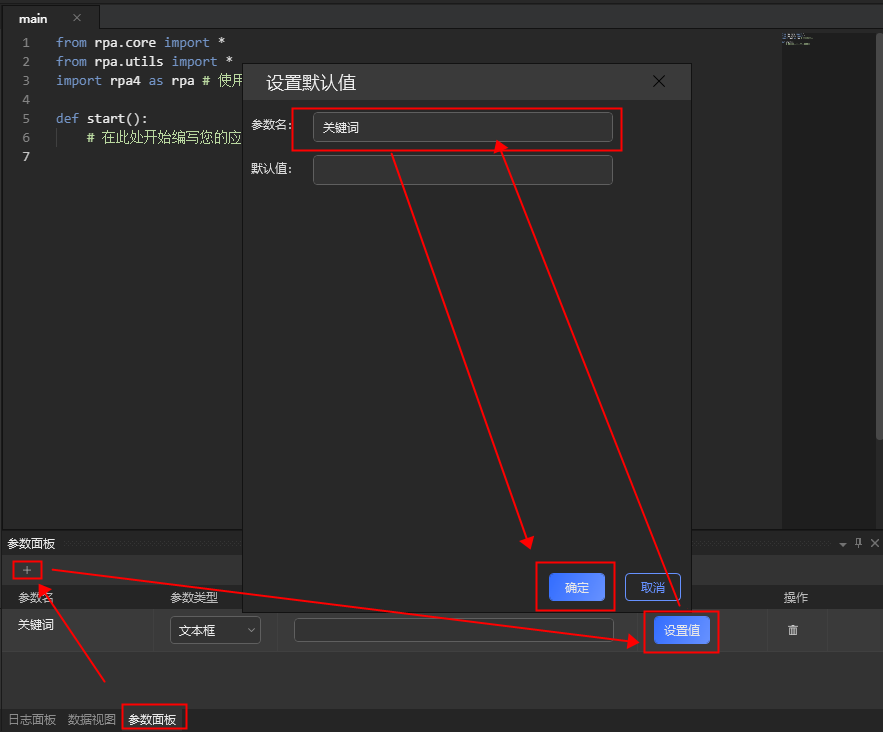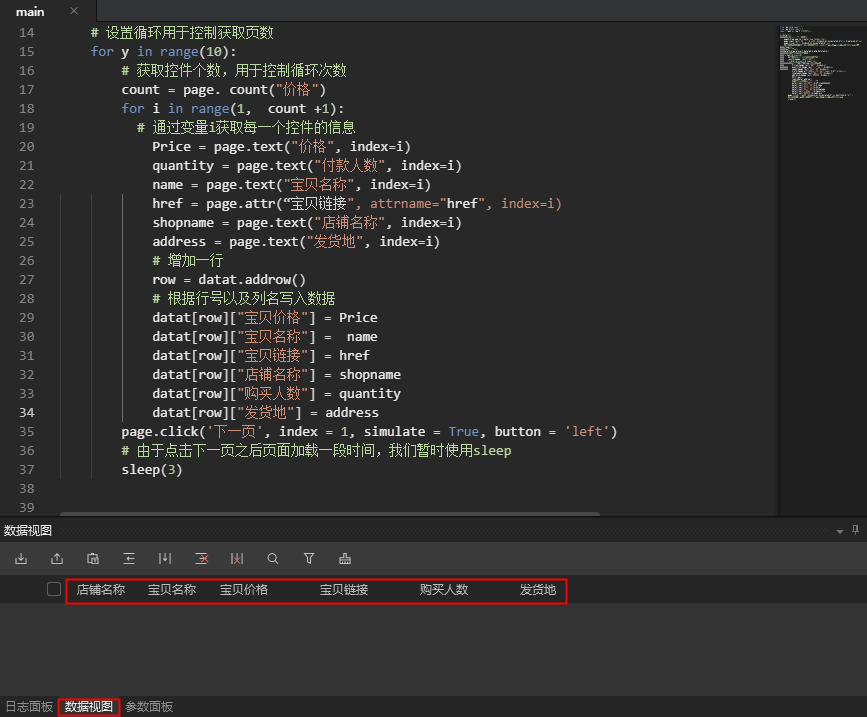API与工具

# 如何操作IE浏览器

## 案例实现

### 1.设置参数面板### 2.设置数据视图### 3.代码编辑

``from rpa.core import *``from rpa.utils import *``import rpa4 as rpa # 使用V4引擎``def start():``   # 在此处开始编写您的应用``    page = rpa.app.ie.create("www.taobao.com")``    page.input_text('输入框', '盆栽', index = 1, simulate = False, replace = True)``    page.click('搜索', index = 1, simulate = True)``    # 此时发现在点击搜索按钮以后页面跳转，要加载一段时间，我们暂时使用sleep测试``    sleep(3)``    # 创建数据视图``    datat = rpa.project.datatable_pkg.Datatable()``    # 设置循环用于控制获取页数``    for y in range(10):``        # 获取控件个数，用于控制循环次数``        count = page. count("价格")``        for i in range(1,  count +1):``          # 通过变量i获取每一个控件的信息``            Price = page.text("价格", index=i)``            quantity = page.text("付款人数", index=i)``            name = page.text("宝贝名称", index=i)``            href = page.attr(“宝贝链接", attrname="href", index=i)``            shopname = page.text("店铺名称", index=i)``            address = page.text("发货地", index=i)``            # 增加一行``            row = datat.addrow()``            # 根据行号以及列名写入数据``            datat[row]["宝贝价格"] = Price        ``            datat[row]["宝贝名称"] =  name``            datat[row]["宝贝链接"] = href``            datat[row]["店铺名称"] = shopname``            datat[row]["购买人数"] = quantity``            datat[row]["发货地"] = address``        page.click('下一页', index = 1, simulate = True, button = 'left')``        # 由于点击下一页之后页面加载一段时间，我们暂时使用sleep``        sleep(3)``

### 4. 录制注意点

• 录制宝贝名称控件时，由于计划使用该控件获取href属性即url，所以要录制到a标签，并非div标签。
• 捕捉相似控件时，若不存在相似控件，则需重新录制控件，以保证控件准确性。
• 修改控件属性时，要修改一次验证一次。